We’re taking a break from our portfolio series and million sample simulations to return to a subject that we haven’t discussed of late despite its featured spot in this blog’s name—options. In this post, we’ll look at the buy-write (BXM) and put-write (PUT) indices on the S&P 500, as conceived, calculated, and published by the CBOE. Note: we’ve discussed the buy-write strategy in the past here and here. In those posts, we analyzed the performance of the buy-write relative to its underlying index, the S&P 500. In this post, we examine the buy-write relative to its close cousin, the put-write.

CBOE’s buy-write index is meant to capture the returns from buying the S&P 500 and simultaneously writing (selling) the closest out-of-the-money (OTM) call with an expiration of close to 30 days. The put-write index sells an at-the-money (ATM) put with close to 30 days to expiration, collateralized by holding Treasury notes with durations between one and three months in length.

While these two indices aren’t exactly the same, they’re very close. To write a covered call one needs to own an equivalent amount of stock, hence the buy-write implies buying 100 “shares” of the index. Similarly, the put-write holds the equivalent dollar value of what would be required to buy the stock if the put were exercised. Due to put-call parity, the value of these two positions should be relatively similar.

Put-call parity is given by the following:
$P + S_{0} = C + Xe^{-rT}$

Where:

$$P$$ = put price; $$S_{0}$$ = stock price at time zero; $$C$$ = call price; and $$Xe^{-rT}$$ = strike price of option discounted by the time to expiration at the prevailing interest rate.

The put price plus the stock price at any time should equal the call price plus the discounted value of the strike price. If not, there’s an arbitrage opportunity. Such a relationship underpins the two indices. The put and call prices should be roughly equivalent except for differences in moneyness (the call is slightly OTM while the put is ATM) and differences in discount rates, which have been modest for most of the time period.1 At trade inception, the puts and calls are similar and the stock and cash are the same value. At expiration, the cash collected on the sale of the options (which should have been nearly equivalent) is offset only by difference between the stock and strike price when the stock is below the strike. Again, similar values.

In between those times, there could be small differences due to market imbalances, but not enough to produce meaningful outperformance. If anything, the buy-write should perform slightly better than the put-write since the call is slightly OTM and the S&P has generally enjoyed an upward bias for most of its history.

Yet that is not what happened. We show the buy-write and put-write indices since inception in the graph below.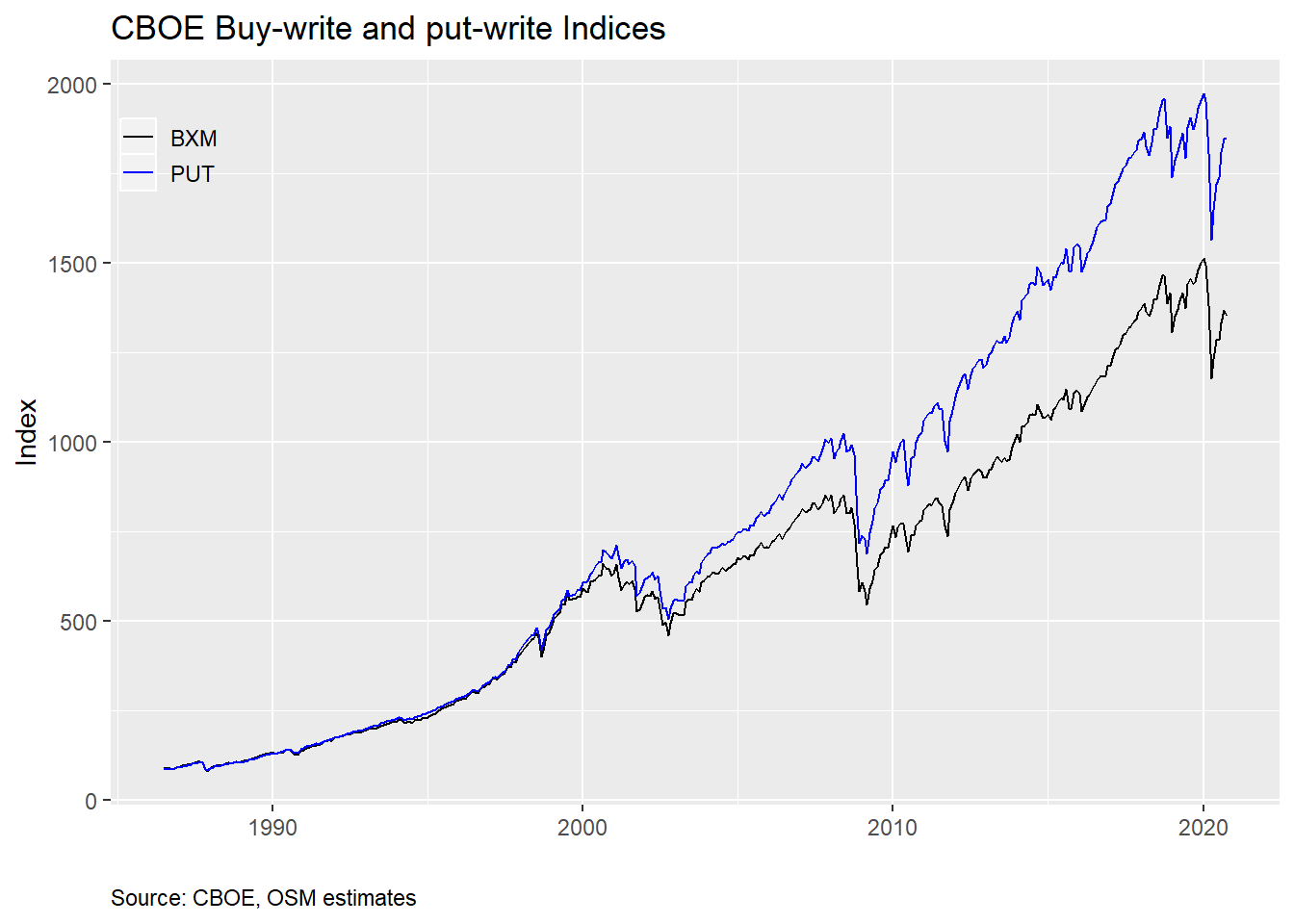The put-write is absolutely crushing it! Since inception, it’s averaged almost 1% points of outperformance per year relative to the buy-write. Since 2000, that outperformance has been closer to 1.4% points. Such performance has come to be known as the BXM-PUT conundrum, and was first discussed by the CBOE in a 2014 whitepaper. AQR Capital Management also discussed the conundrum in one of its papers. Despite this coverage, not much seems to have been written on the topic since. Google Scholar shows only 4 citations for the AQR paper.

Why such little coverage despite what appears to be a huge anomaly, at least according to the graph? Let’s look at annual returns to tease this out.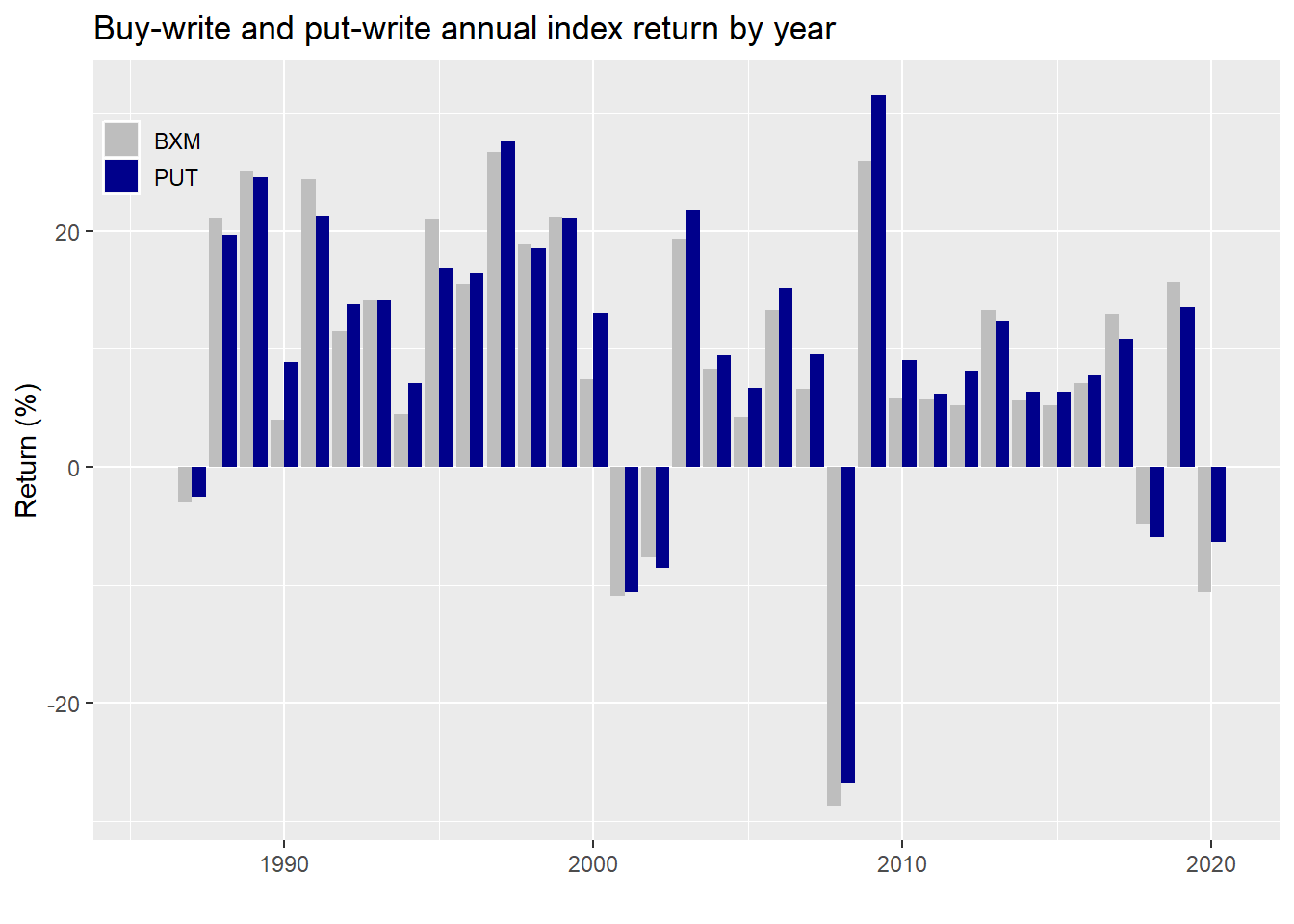As one can see, the put-write index outperforms the buy-write in most years, about 68% of the time. According to the authors, potential reasons why this divergence might occur include differences in risk-free returns, leverage, the options’ underlying sensitivity to changes in the index (known as delta), the observation that put prices are rich relative to calls for similar out-of-the-moneyness (known ans skew), or how the indices are constructed. Rather than explain all these, we’ll skip to the punchline: according to the different authors, differences in construction have the highest explanatory power.

Without getting into the detail of index option settlement pricing or how the authors constructed the tests to arrive at their conclusions, the discovery was that the buy-write is exposed to the S&P500 for about four hours longer per month than the put-write on expiration days. Recall, the options are written on monthly contracts. When the contract expires, the buy-write is theoretically still holding the S&P, while the put-write has no market exposure. And, as it so happens, those four hours at the monthly expirations generated a negative return in the S&P relative to the settlement price of the options in the period of study.

This clearly begs the question, if there is no economic or mechanical reason for this anomaly, why should it persist? Presumably, it shouldn’t, at least not according to finance theory.2 If there is indeed an inexplicable negative return during this four hour period, why aren’t investors exploiting it?

Indeed it appears the market may have actually done just that. Here’s the cumulative monthly return for the two indices from the June 2004 to December 2014, the same time period in which the CBOE paper argues that construction differences do the best job in explaining the conundrum.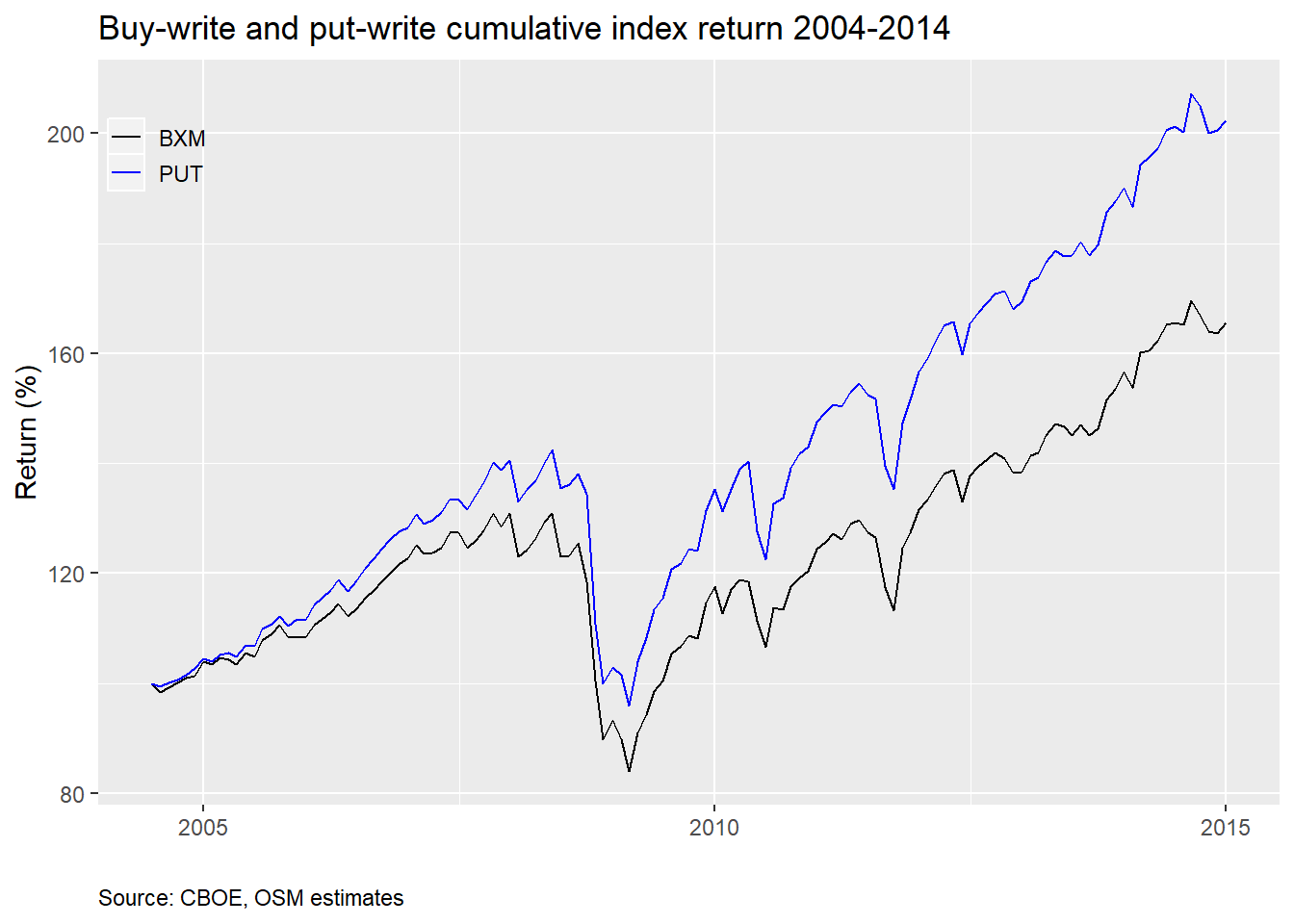Now here’s the period since the paper was published.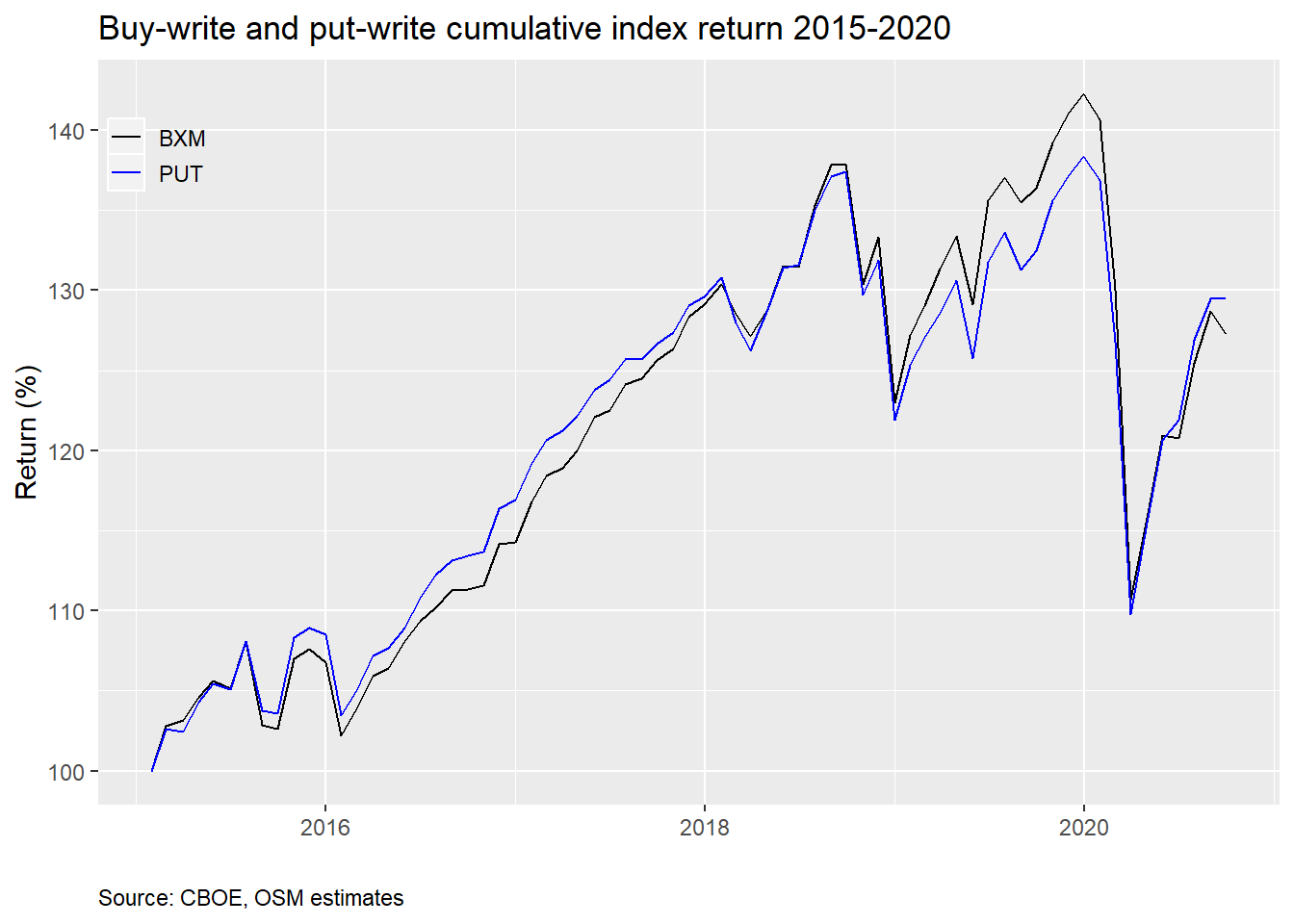Looks like the conundrum may have been arbitraged away! The cumulative returns of both indices now track each other much better. However, there does appear to be a divergence in the 2019 period when the buy-write actually outperforms the put-write. Recall, 2019 was a great year for the market, so the fact that the buy-write calls are slightly OTM might explain this performance. Whatever the case, the average monthly outperformance of the put-write erodes meaningfully as shown by the table below.

Table 1: Monthly average put-write outperformance pre- and post-2015
BeforeAfterP-value
0.15%0.014%0.09

Even though the differences in average outperformance may not qualify as significant using traditional statistical measures like the p-value, they seem pretty stark to us.

We’ve been using month end pricing for the analysis, as that is how most of the long-term publicly available data is recorded. This periodicity may accentuate the results, so let’s look at daily data. Unfortunately, there’s only a full data set starting from 2007.

Below we graph the cumulative difference in daily returns to the put-write vs. buy-write indices. This is effectively the return to going long the put-write and short the buy-write.We see positive returns until about 2014-2015, after which there’s little movement in the long-short portfolio until 2017, when returns go in the opposite direction only to reverse themselves in 2020. Let’s zoom in on that period.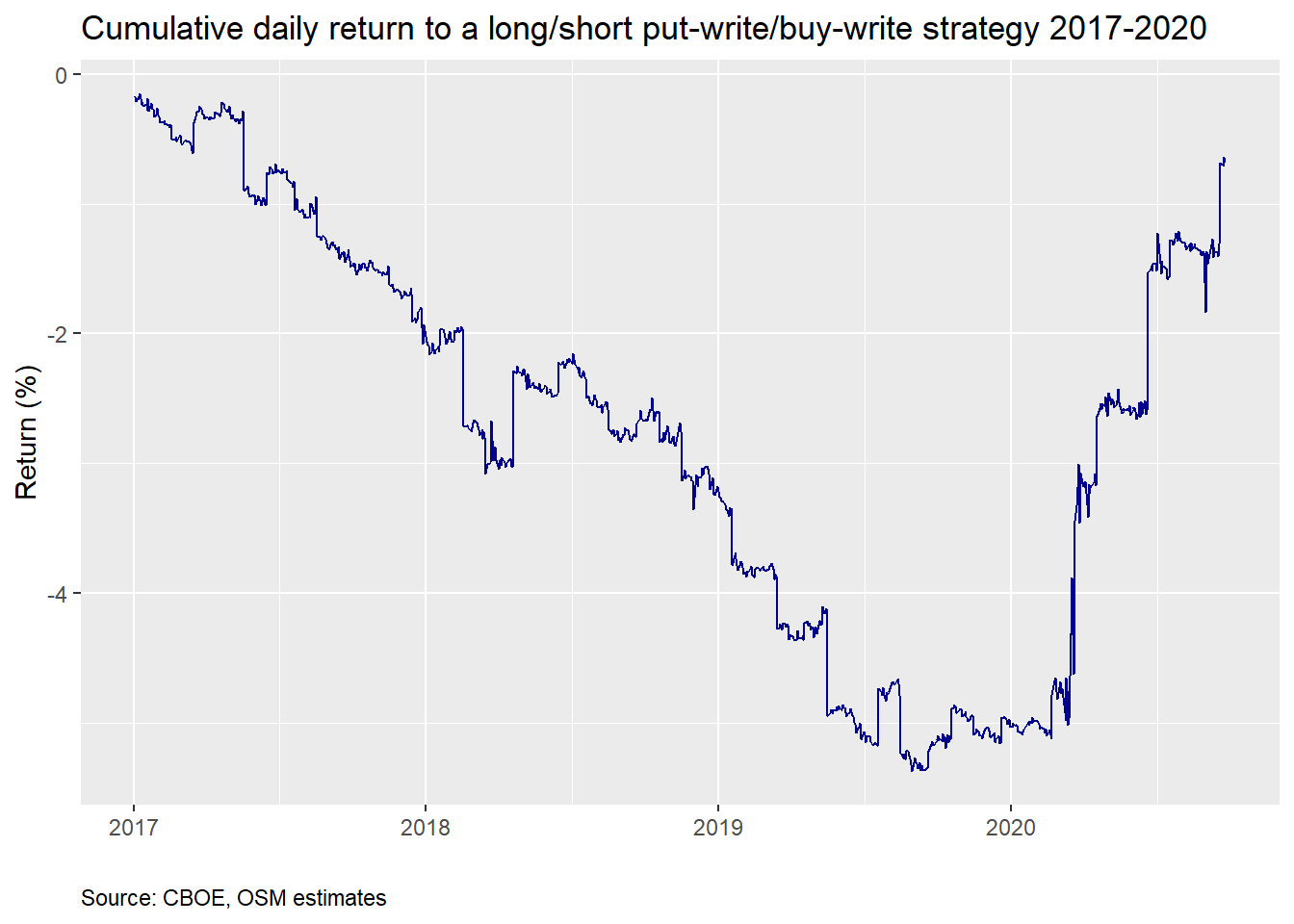The 2017-2020 period is one of pretty consistent negative returns. It only reverses in 2020 at the onset of the covid-19 crisis, which of course saw a jump in volatility with the huge downdraft in the market. Perhaps the construction differences are once again playing a role. Bear markets aren’t usually friendly to arbitrage strategies that rely on stable historical relationships. Indeed, when we remove option expirations from the graph, as was done in the AQR paper, the cumulative return is de minimis; it oscillates between zero and 0.5%. Those four pesky hours are generating all the return!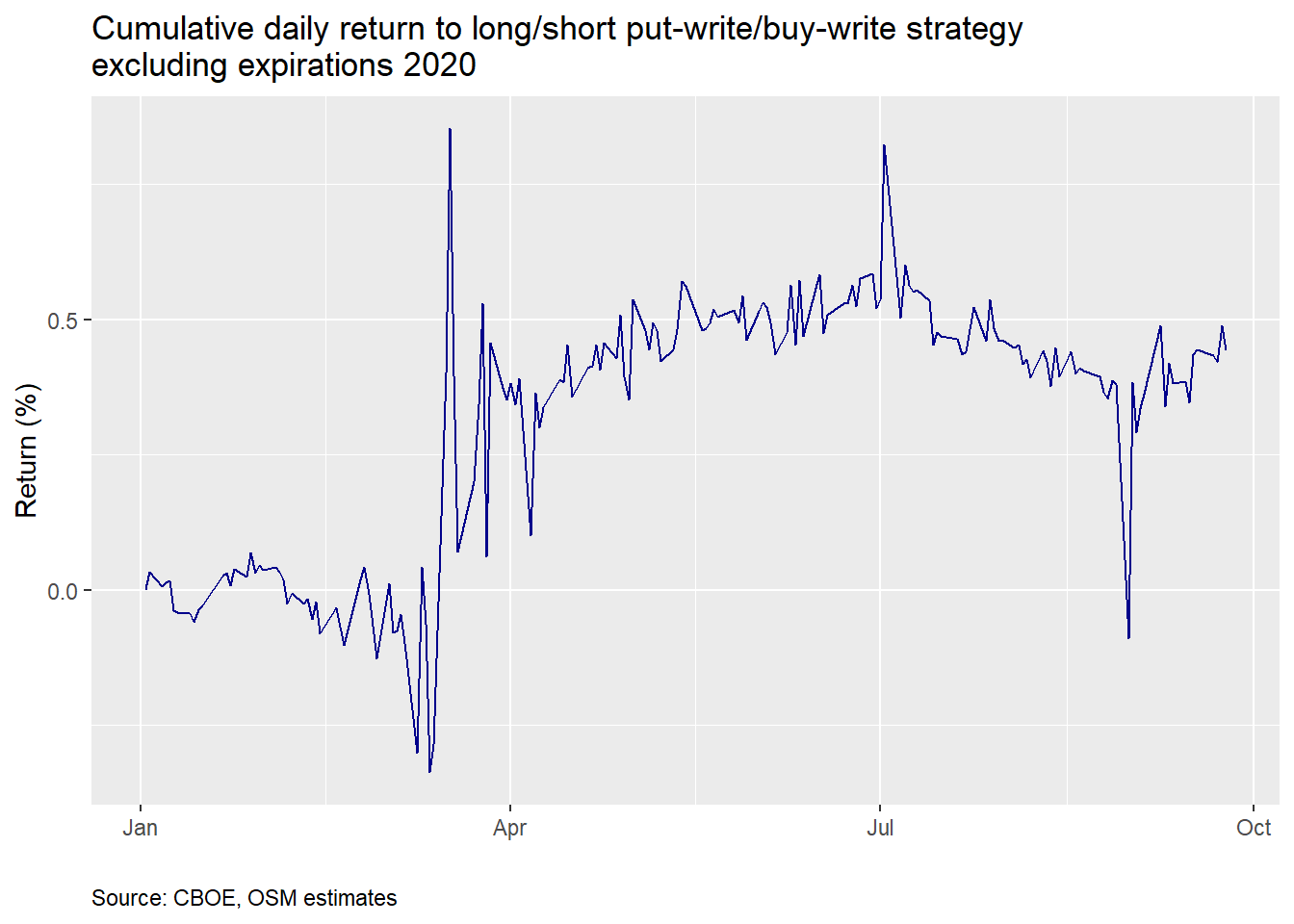What’s the takeaway? Next time there’s a bear market short the buy-write against the put-write? Not so sure about that and this blog doesn’t offer investment advice. What we do think is interesting is that there appears to have been an expiration anomaly prior to the CBOE white paper that then disappeared afterward. If you’ve discovered an anomaly don’t tell anyone! Unless, of course, to exploit that anomaly you need others to buy so you can sell, in which case you should get loud and proud on your favorite media outlet.

The second interesting point is that the anomaly has returned. While we conjectured it may be due to lack of arbitrageurs, there could be other reasons we’re not aware of. Whether or not it will persist is an open question we’ll save for another time. Until then, and before we return to our portfolio series, the Python and R code are below. Enjoy!

#### Python

# Built using Python 3.7.4

import pandas as pd
import numpy as np
import matplotlib.pyplot as plt
%matplotlib inline

# Note: only goest to 2019-06-28
cboe = cboe.iloc[:397,]

# Clean columns
import re
col_names = ['date'] + [x.lower() for x in cboe.columns.to_list() if x != 'Unnamed: 0']
col_names = [re.sub(r'[^\w]', '', x) for x in col_names]
col_names = [x.replace("sm","") if "sm" in x else x for x in col_names]
cboe.columns = col_names
cboe['date'] = pd.to_datetime(cboe['date'])

non_nums = ['sptr', 'vix', 'bxy']
cboe[non_nums] = cboe[non_nums].apply(pd.to_numeric, errors='coerce', axis=1)

cboe = cboe.loc[:, ['date', 'spx', 'sptr', 'vix', 'bxm', 'put']]

# Retrieve near term data
# Pulled more data than used.

bxm['Date'] = pd.to_datetime(bxm['Date'])
bxm.columns = ['date', 'price']

put['Unnamed: 0'] = pd.to_datetime(put['Unnamed: 0'])
put = put.iloc[:,:2]
put.columns = ['date', 'price']

vix['Date'] = pd.to_datetime(vix['Date'])
vix = vix.loc[:,['Date', 'VIX Close']]
vix.columns = ['date', 'price']

spx = spx.reset_index()
spx.columns = ['date', 'price']

sptr = sptr.reset_index()
sptr.columns = ['date', 'price']

# Was struggling to figure out how to bring all these dataframes together. Found the solution
# on SO:
# https://stackoverflow.com/questions/23668427/pandas-three-way-joining-multiple-dataframes-on-columns

prices.columns = ['date','spx', 'sptr', 'vix', 'bxm', 'put']
prices.set_index('date', inplace=True)

prices_mon = prices.resample('M').last()

cboe.set_index('date', inplace=True) # For ease of indexing
cboe = cboe[:'2019-04-30'].append(prices_mon['2019-05-31':]) # Remove overlaps

## EDA
# Graph BXM & PUT
plt.style.use('ggplot')

cboe[['bxm','put']].plot(color = ['grey', 'darkblue'], figsize=(12,6))
plt.xlabel("")
plt.ylabel("Index")
plt.legend(['BXM', 'PUT'])
plt.show()

# Graph annual returns by year
from functools import reduce
dfs = [spx, sptr, vix, bxm, put]
prices = reduce(lambda left, right: pd.merge(left, right, how = 'left', on = 'date'), dfs)

diffs = (ret_by_year['put'] - ret_by_year['bxm']).values/100
stats.ttest_1samp(diffs[1:],0.00)

# Analyze differences in return before and after 2015
from scipy import stats
returns = cboe[['bxm', 'put']].pct_change()
diff = returns['put'] - returns['bxm']

before = diff['2004-06-01':'2014-12-31']
after = diff['2015-01-01':]
t_test = stats.ttest_ind(before, after, equal_var=False)

print(f'Mean before 2015: {np.mean(before):0.03}')
print(f'Mean after 2015: {np.mean(after):0.03}')
print(f'P-value: {t_test:0.03f}')

# Graph before 2015
((1+returns['2004-06-1':'2014-12-31']).cumprod()*100).plot(color = ['darkblue', 'grey'], figsize=(12,6))
plt.xlabel("")
plt.ylabel("Retrun (%)")
plt.title("Buy-write and put-write ETFS cumulative return")
plt.show()

# Graph after 2015
((1+returns['2014-01-01':]).cumprod()*100).plot(color = ['darkblue', 'grey'], figsize=(12,6))
plt.xlabel("")
plt.ylabel("Retrun (%)")
plt.title("Buy-write and put-write ETFS cumulative return")
plt.show()

## Create daily return df
daily = pd.merge(put, bxm, how = "left", on = 'date')
daily.set_index('date', inplace=True)
daily.columns = ['put', 'bxm']
daily_return = daily.pct_change()
daily_return['diff'] = daily_return['put'] - daily_return['bxm']

# Graph daily return
(((1+daily_return['diff']).cumprod()-1)*100).plot(color='darkblue', figsize=(12,6))
plt.xlabel("")
plt.ylabel("Return (%)")
plt.show()

# Graph daily return since 2017
(((1+daily_return.loc["2017-01-01":, 'diff']).cumprod()-1)*100).plot(color='darkblue', figsize=(12,6))
plt.xlabel("")
plt.ylabel("Return (%)")
plt.show()

# Remove expiration days 2020
exp_dates = pd.to_datetime(np.array(["2020-01-17", "2020-02-21", "2020-03-20",
"2020-04-17", "2020-05-15", "2020-06-19",
"2020-07-17", "2020-08-21", "2020-09-18"]))
daily_ret_20 = daily_return['2020-01-01':]
daily_ret_20 = daily_ret_20.drop(exp_dates)

# Graph cumulative daily return excluding expiration days 2020
(((1+daily_ret_20['diff']).cumprod()-1)*100).plot(color = 'darkblue', figsize=(12,6))
plt.xlabel("")
plt.ylabel("Return (%)")
plt.show()

#### R

# Built using R 3.6.2

suppressPackageStartupMessages({
library(tidyquant)
library(tidyverse)
})

# Get data and process

cboe <- cboe %>%
rename("date" = ...1,
"BXM" = BXMSM,
"BXY" = BXYSM,
"CLL" = CLL*,
"VIX" = VIX®,
"PUT" = PUTSM) %>%
mutate(date = ymd(date))

colnames(cboe) <- tolower(colnames(cboe))

bxm <- read_csv(bxm_url, skip = 3)
bxm <- bxm %>%
mutate(Date = mdy(Date)) %>%
select(Date, BXM Level) %>%
rename("date" = Date,
"price" = BXM Level)

put <- read_csv(put_url, skip = 6)
put <- put %>%
mutate(X1 = mdy(X1)) %>%
select(X1, Daily Closing Prices) %>%
rename("date" = X1,
"price" = Daily Closing Prices)

vix <- vix %>%
mutate(Date = mdy(Date)) %>%
select(Date, VIX Close) %>%
rename("date" = Date,
"price" = VIX Close)

spx <- getSymbols("^GSPC", src = "yahoo", from = "2004-01-01", auto.assign = FALSE) %>%
colnames<-("price")

sptr <- getSymbols("^SP500TR", src = "yahoo", from = "2004-01-01", auto.assign = FALSE) %>%
colnames<-("price")

put_xts <- as.xts(put$price, order.by = put$date)
vix_xts <- as.xts(vix$price, order.by = vix$date)
bxm_xts <- as.xts(bxm$price, order.by = bxm$date)

prices <- merge(spx, sptr, vix_xts, bxm_xts, put_xts)
tail(prices)
colnames(prices) <- c('spx', 'sptr', 'vix', 'bxm', 'put')

price_mon <- to.monthly(prices, indexAt = 'lastof', OHLC = FALSE)

nrow(price_mon)

price_bind <- price_mon["2019-05-31/2020-12-31"]

price_df <- data.frame(date = index(price_bind), coredata(price_bind))

cboe <- cboe %>%
filter(date <= '2019-04-30') %>%
select(date, spx, sptr, vix, bxm, put) %>%
bind_rows(price_df)

saveRDS(cboe,'cboe_bxm_put.rds')

## Graph
cboe %>%
select(date, bxm, put) %>%
gather(key,value, -date) %>%
ggplot(aes(date,value, color = key)) +
geom_line() +
scale_color_manual("", labels = c('BXM', 'PUT'), values = c("black", "blue")) +
labs(x= '',
y = "Index",
title = "Buy-write and Put-write Indices",
caption = "Source: CBOE, OSM estimates")+
theme(legend.position = c(0.05,0.9), legend.key.size = unit(.5, "cm"),
legend.background = element_rect(fill = NA),
plot.caption = element_text(hjust = 0))

## Data
mean_all <- cboe %>%
mutate(year = year(date)) %>%
group_by(year) %>%
summarise_at(vars('bxm', 'put'), last) %>%
mutate_at(vars('bxm', 'put'), function(x) x/lag(x)-1) %>%
mutate(diff = put - bxm) %>%
# filter(year <= 2015) %>%
summarise(mean = mean(diff, na.rm = TRUE)) %>%
as.numeric()

mean_2000 <- cboe %>%
mutate(year = year(date)) %>%
group_by(year) %>%
summarise_at(vars('bxm', 'put'), last) %>%
mutate_at(vars('bxm', 'put'), function(x) x/lag(x)-1) %>%
mutate(diff = put - bxm) %>%
filter(year >= 2000) %>%
summarise(mean = mean(diff, na.rm = TRUE)) %>%
as.numeric()

# Annual return by year graph
cboe %>%
mutate(year = year(date)) %>%
group_by(year) %>%
summarise_at(vars('bxm', 'put'), last) %>%
mutate_at(vars('bxm', 'put'), function(x) x/lag(x)-1) %>%
gather(key, value, -year) %>%
ggplot(aes(year, value*100, fill = key)) +
geom_bar(stat = 'identity', position = 'dodge') +
scale_fill_manual("", labels = c('BXM', 'PUT'), values = c('grey', 'darkblue')) +
theme(legend.position = c(0.05,0.9), legend.key.size = unit(.5, "cm"),
legend.background = element_rect(fill = NA)) +
labs(x = '',
y = 'Return (%)',
title = 'Annual return by year')

# Frequency of outperformance
freq_opf <- cboe %>%
mutate(year = year(date)) %>%
group_by(year) %>%
summarise_at(vars('bxm', 'put'), last) %>%
mutate_at(vars('bxm', 'put'), function(x) x/lag(x)-1) %>%
summarise(mean =mean(put > bxm, na.rm = TRUE)) %>%
as.numeric() %>%
round(.,2)*100

# Cumulative returns matching paper date
cboe %>%
select(date, bxm, put) %>%
gather(key,value, -date) %>%
group_by(key) %>%
filter(date >= "2004-06-01", date <= "2014-12-31") %>%
mutate(value = value/first(value)) %>%
ggplot(aes(date,value*100, color = key)) +
geom_line() +
scale_color_manual("", labels = c('BXM', 'PUT'), values = c("black", "blue")) +
labs(x= '',
y = "Return (%)",
title = "Buy-write and Put-write cumulative return",
caption = "Source: CBOE, OSM estimates")+
theme(legend.position = c(0.05,0.9), legend.key.size = unit(.5, "cm"),
legend.background = element_rect(fill = NA),
plot.caption = element_text(hjust = 0))

# Graph after 2014
cboe %>%
select(date, bxm, put) %>%
gather(key,value, -date) %>%
group_by(key) %>%
filter(date > "2014-12-31") %>%
mutate(value = value/first(value)) %>%
ggplot(aes(date,value*100, color = key)) +
geom_line() +
scale_color_manual("", labels = c('BXM', 'PUT'), values = c("black", "blue")) +
labs(x= '',
y = "Return (%)",
title = "Buy-write and Put-write cumulative return",
caption = "Source: CBOE, OSM estimates")+
theme(legend.position = c(0.05,0.9), legend.key.size = unit(.5, "cm"),
legend.background = element_rect(fill = NA),
plot.caption = element_text(hjust = 0))

## Before and after publish date
before <- cboe %>%
mutate_at(vars('bxm', 'put'), function(x) x/lag(x)) %>%
mutate(diff = put - bxm) %>%
filter(date >= "2004-06-01", date <= "2014-12-31") %>%
select(diff) %>%
drop_na() %>%
unlist() %>%
as.numeric()

after <- cboe %>%
mutate_at(vars('bxm', 'put'), function(x) x/lag(x)) %>%
mutate(diff = put - bxm) %>%
filter(date > "2014-12-31") %>%
select(diff) %>%
drop_na() %>%
unlist() %>%
as.numeric()

## Table
data.frame(Before = paste(round(mean(before),4)*100, "%", sep=""),
After = paste(round(mean(after),5)*100, "%", sep=""),
P-value = round(t.test(before, after)\$p.value,2),
check.names = FALSE) %>%
knitr::kable(caption ="Monthly average put-write outperformance pre- and post-2015",
align = "r")

## Daily
daily <- put %>%
left_join(bxm, by = 'date') %>%
colnames<-(c("date", "put", "bxm"))

daily %>%
mutate_at(vars('put', 'bxm'), function(x) x/lag(x)-1) %>%
drop_na() %>%
mutate(diff = put-bxm) %>%
mutate(cum_diff = cumprod(1 + diff)-1) %>%
ggplot(aes(date, cum_diff*100)) +
geom_line(color='darkblue') +
labs(x="",
y = "Return (%)",
caption = "Source: CBOE, OSM estimates") +
theme(plot.caption = element_text(hjust=0))

# Daily from 2015 on
cboe %>%
select(date, spx, bxm, put) %>%
gather(key,value, -date) %>%
group_by(key) %>%
filter(date >= "2014-12-31") %>%
mutate(value = value/first(value)) %>%
ggplot(aes(date,value*100, color = key)) +
geom_line() +
scale_color_manual("", labels = c('BXM', 'PUT', 'SP500'),
values = c("black", "blue", "purple")) +
labs(x= '',
y = "Return (%)",
title = "Buy-write and Put-write cumulative return",
caption = "Source: CBOE, OSM estimates")+
theme(legend.position = c(0.05,0.9), legend.key.size = unit(.5, "cm"),
legend.background = element_rect(fill = NA),
plot.caption = element_text(hjust = 0))

## Exclude expirations
exp_dates = c("2020-01-17", "2020-02-21", "2020-03-20",
"2020-04-17", "2020-05-15", "2020-06-19",
"2020-07-17", "2020-08-21", "2020-09-18")

daily %>%
mutate_at(vars('put', 'bxm'), function(x) x/lag(x)-1) %>%
drop_na() %>%
mutate(diff = put-bxm) %>%
filter(date >= "2020-01-01") %>%
filter(!date %in% as.Date(exp_dates,"%Y-%m-%d")) %>%
mutate(cum_diff = cumprod(1 + diff)-1) %>%
ggplot(aes(date, cum_diff*100)) +
geom_line(color='darkblue') +
labs(x="",
y = "Return (%)",
theme(plot.caption = element_text(hjust=0))